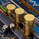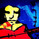8455 lượt xem
I have converted my volatility based force scalper into strategy. Nice to see it is so profitable. Work best with Heikin Ashi bar.
```//@version=2
strategy("yuthavithi volatility based force trade scalper strategy", overlay=true)

fast = input(3, minval= 1, title="Fast")
slow = input(20, minval = 1, title = "Slow")
atrFast = input(20, minval = 1, title = "ATR Fast")
atrSlow = input(50, minval = 1, title = "ATR Slow")

len = input(20, minval=1, title="Length")
multiplier = input(2, minval=1, title="multiplier")
src = input(close, title="Source")
bbMid = sma(src, len)
plot(bbMid, color=blue)

atrFastVal = atr(atrFast)
atrSlowVal = atr(atrSlow)
stdOut = stdev(close, len)
bbUpper = bbMid + stdOut * multiplier
bbLower = bbMid - stdOut * multiplier
plot(bbUpper, color = (atrFastVal > atrSlowVal ? red : silver))
plot(bbLower, color = (atrFastVal > atrSlowVal ? red : silver))

force = volume * (close -  nz(close))
xforce = cum(force)
xforceFast = ema(xforce, fast)
xforceSlow = ema(xforce, slow)

bearish = ((xforceFast < xforceSlow) and (atrFastVal > atrSlowVal)) and ((xforceFast > xforceSlow) or (atrFastVal < atrSlowVal)) and (close < open)
bullish = ((xforceFast > xforceSlow) and (atrFastVal > atrSlowVal)) and ((xforceFast < xforceSlow) or (atrFastVal < atrSlowVal)) and (close > open)

if (bullish)